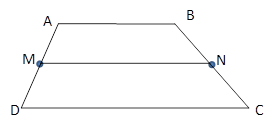Chapter 4.4, Problem 11E### Elementary Geometry for College St...

6th Edition
Daniel C. Alexander + 1 other
ISBN: 9781285195698

#### Solutions

Chapter
Section### Elementary Geometry for College St...

6th Edition
Daniel C. Alexander + 1 other
ISBN: 9781285195698
Textbook Problem
15 views

# In Exercises 11 to 16, the drawing shows trapezoid ABCD with A B ¯ | | D C ¯ ; also, M and N are midpoints of A D ¯ and B C ¯ , respectively.Exercises 11-16Given: A B   =   7.3   a n d   D C   =   12.1 Find: M N

To determine

To Find:

MN of trapezoid ABCD.

Explanation

Consider the following trapezoid ABCD.

Given:

AB = 7.3 and DC = 12.1

In the given trapezoid, AB¯||DC¯

### Still sussing out bartleby?

Check out a sample textbook solution.

See a sample solution

#### The Solution to Your Study Problems

Bartleby provides explanations to thousands of textbook problems written by our experts, many with advanced degrees!

Get Started

#### In Exercises 13-20, sketch a set of coordinate axes and plot each point. 13. (2, 5)

Applied Calculus for the Managerial, Life, and Social Sciences: A Brief Approach

#### Find the remaining trigonometric ratios. 32. cosx=13,x32

Single Variable Calculus: Early Transcendentals, Volume I

#### In Exercises 516, evaluate the given quantity. log1,000

Finite Mathematics and Applied Calculus (MindTap Course List)

#### Find the limit. limh0(h1)3+1h

Single Variable Calculus: Early Transcendentals

#### An integral for the solid obtained by rotating the region at the right about the y-axis is:

Study Guide for Stewart's Single Variable Calculus: Early Transcendentals, 8th